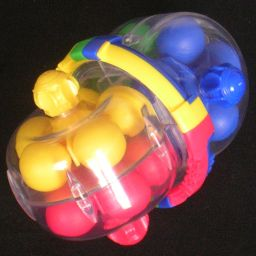# Super Brain SpinnerThe Super Brain Spinner is a puzzle consisting of four coloured wheels, each with 5 balls of a matching colour. One half has the blue and the green wheel on the same axis, but which can rotate independently by turning the knobs on the outside of the transparent casing. The other half is similar, and has a yellow and a red wheel on one axis. The two halves of the puzzle can be twisted relative to each other until the two axes are parallel, and the four wheels lie in two parallel planes. When you then turn any wheel, all 10 balls in the two wheels in that plane move around in a loop. It therefore has two loops of ten balls. By turning over one half of the puzzle, you switch two wheels between loops, mixing them up. You can also twist one half of the puzzle a quarter turn, and in this configuration all 20 balls form a single long loop, and turning any wheel causes all the wheels to move.

The Super Brain Spinner is marketed by Foxmind, and invented by DieN (Dmitry Novikov). He also invented the Turbo Mind Twister.

## The number of positions:

There are 20 balls, 5 of each colour. That gives 20! / 5!4 = 11,732,745,024 possible positions of the balls, relative to the wheels. The two halves of the puzzle have 4 positions relative to each other, so if you consider those to be distinct then there are 4 times as many positions, namely 46,930,980,096.

## Solution:

Phase 1: Solve the Blue wheel.

1. Hold the puzzle so that the blue wheel lies at the top of the left hand side of the puzzle. Make sure all the wheels are horizontal, parallel to each other.
2. Find a blue ball that does not lie in the blue wheel.
3. If the ball lies in the top wheel on the right hand side, then turn over the right half to bring it to the bottom.
4. Move the bottom loop until the blue ball is at the front, directly to the right of the middle seam.
5. Turn over the right half to bring it to the top.
6. Move the top loop anti-clockwise one step, inserting the blue ball into the blue wheel.
7. Repeat steps b-f until the blue wheel is solved.

Phase 2: Solve the Yellow wheel.

1. Hold the puzzle so that the blue wheel lies at the bottom of the left hand side of the puzzle. Make sure all the wheels are horizontal, parallel to each other.
2. Make sure the yellow wheel lies at the bottom of the right hand side, turning over the right half if necessary to make it so.
3. Move the top loop until there is a yellow ball at the front, directly to the left of the middle seam.
4. Turn over the right half to bring the yellow wheel to the top.
5. Move the top loop anti-clockwise one step, inserting the yellow ball into the yellow wheel.
6. Turn over the right half to bring the yellow wheel to the bottom.
7. Repeat steps b-f until the yellow wheel is solved.

Phase 3: Solve the Red and Green wheels.

1. Hold the puzzle so that the red and the green wheel lie at the top.
2. Below I'll describe a move sequence that can swap any two non-adjacent balls in the top loop. Examine the loop of balls to see which two balls can be swapped to improve the situation, usually by putting balls of the same colour closer together.
3. Having chosen two non-adjacent balls to swap, turn the top loop so that one ball is in the left wheel, while the other is at the front, just to the right of the front seam.
4. Do the following 11 move sequence:
1. Turn over the right hand half of the puzzle, bringing one of the chosen balls to the bottom.
2. Move the loop anti-clockwise, until the other ball passes the seam at the front and enters the right wheel.
3. Turn over the right hand half of the puzzle.
4. Move the loop clockwise, one step.
5. Turn over the right hand half of the puzzle.
6. Move the loop clockwise, one step.
7. Turn over the right hand half of the puzzle.
8. Move the loop anti-clockwise, one step.
9. Turn over the right hand half of the puzzle.
10. Move the loop clockwise, until all the yellow balls are back in the yellow wheel on the right.
11. Turn over the right hand half of the puzzle.
Using the straightforward notation where U and R stand for the two kinds of move, and x for the number of balls lying between the two balls you want to swap, the move sequence is:
R U-(x+1) R U1 R U1 R U-1 R Ux R
5. Repeat b-d until the red and yellow balls in the top loop are separated.
6. Move the top loop until all the balls are in their matching wheels.
7. Twist the ring on the seam to match the left hand side wheel colours.
8. Twist the right hand half of the puzzle to match the colours on the ring on the seam.